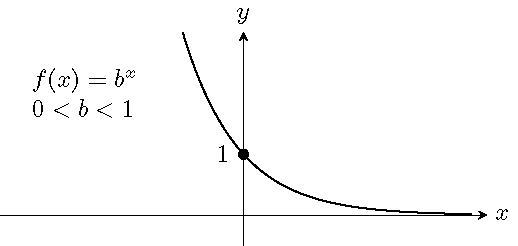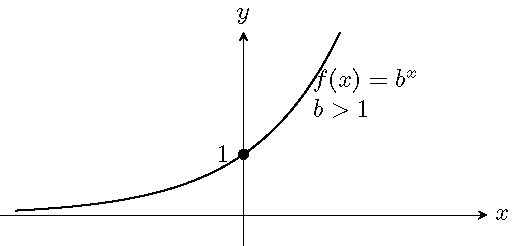# Topic 16 Exponential Functions

## 16.1 Half-life

Half-life is the time required for a quantity to reduce to half of its initial value.

A certain pesticide is used against insects. The half-life of the pesticide is about 12 days. After a month how much would left if the initial amount of the pesticide is 10 g? Can you write a function for the remaining quantity of the pesticide after $$t$$ days?

More examples on exponential functions can be found on http://passyworldofmathematics.com/exponents-in-the-real-world/.

## 16.2 Definition and Graphs of Exponential Functions

Let $$b$$ be a positive number other than $$1$$ (i.e. $$b>0$$ and $$b\neq 1$$). The exponential function $$f$$ of $$x$$ with the base $$b$$ is defined as $f(x)=b^x\quad\quad\text{or}\quad\quad y=b^x.$

Graphs of exponential functions:The exponential function $$f(x)=b^x$$ is a one-to-one function: any vertical line or any horizontal line crosses the graph at most once. Equivalently, the equation $$b^x=c$$ has at most one solution for any real number $$c$$.

## 16.3 The Natural Number $$e$$

The natural number $$e$$ is the number to which the quantity $$\left(1+\dfrac1n\right)^n$$ approaches as $$n$$ takes on increasingly large values. Approximately, $$e\approx2.718281827$$.

## 16.4 Compound Interests

After $$t$$ years, the balance $$A$$ in an account with a principal $$P$$ and annual interest rate $$r$$ is given by the following formulas:

1. For $$n$$ compounding periods per year: $$A=P\left(1+\dfrac{r}{n}\right)^{nt}$$.
2. For compounding continuously: $$A=Pe^{rt}$$.

Example 16.1 A sum of $$\10,000$$ is invested at an annual rate of $$8\%$$, Find the balance, to the nearest hundredth dollar, in the account after $$5$$ years if the interest is compounded

1. monthly,
2. quarterly,
3. semiannually,
4. continuous.

Solution.

1. Find values of $$P$$, $$r$$, $$t$$ and $$n$$. In this case, $$P=10,000$$, $$r=8\%=0.08$$, $$t=5$$ and $$n$$ depends compounding.
2. Plug the values in the formula and calculate.
3. Monthly’’ means $$n=12$$. Then $A=10000\left(1+\frac{0.08}{12}\right)^{5\cdot 12}\approx 14898.46.$
4. Quarterly’’ means $$n=4$$. Then $A=10000\left(1+\frac{0.08}{4}\right)^{5\cdot 4}\approx 14859.47.$
5. semiannually’’ means $$n=2$$. Then $A=10000\left(1+\frac{0.08}{2}\right)^{5\cdot 2}\approx 14802.44.$
6. For continuously compounded interest, we have $A=10000e^{0.08\cdot 5}\approx 14918.25.$

In the compounded investment module, the $$\frac rn$$ is an approximation of the period interest rate. Indeed, if the period rate $$p$$ satisfies the equation $$(1+p)^n=1+r$$, or equivalently $$p=\sqrt[n]{1+r} - 1$$. Using the formula $$(1+x)^n=1+nx+\frac{n(n-1)}{2}x^2+\cdots +x^n$$, one may approximately replace $$1+r$$ by $$(1+\frac rn)$$ and obtain the approximation $$p\approx \frac rn$$.

Example 16.2 The population of a country was about 0.78 billion in the year 2015, with an annual growth rate of about 0.4%. The predicted population is $$P(t)=0.78(1.004)^t$$ billions after $$t$$ years since 2015. To the nearest thousandth of a billion, what will the predicted population of the country be in 2030?

Solution.

The population is approximately $P(15)=0.78(1.004)^{15}\approx 0.828 \quad \text{billions}.$

## 16.5 Practice

Problem 16.1 The value of a car is depreciating according to the formula: $$V=25000(3.2)^{-0.05x}$$, where $$x$$ is the age of the car in years. Find the value of the car, to the nearest dollar, when it is five years old.

Problem 16.2 A sum of \$20,000 is invested at an annual rate of 5.5%, Find the balance, to the nearest dollar, in the account after 5 years subject to

1. monthly compounding,
2. continuously compounding.

Problem 16.3 Sketch the graph of the function and find its range.

1. $$f(x)=3^x$$
2. $$f(x)=\left(\frac13\right)^x$$

Problem 16.4 Use the given function to compare the values of $$f(-1.05)$$, $$f(0)$$ and $$f(2.4)$$ and determine which value is the largest and which value is the smallest. Explain your answer.

1. $$f(x)=\left(\frac{5}{2}\right)^x$$
2. $$f(x)=\left(\frac23\right)^x$$